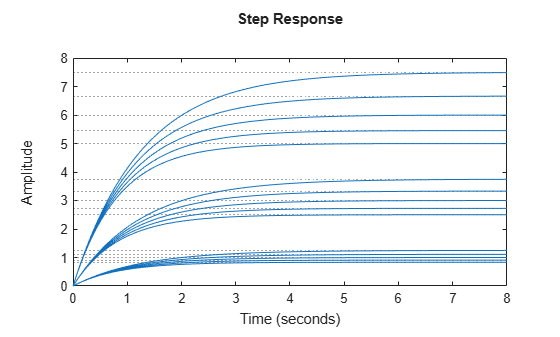Documentation

# sampleBlock

Sample Control Design blocks in generalized model

## Syntax

``Msamp = sampleBlock(M,name,vals)``
``Msamp = sampleBlock(M,nameset,valset)``
``Msamp = sampleBlock(M,nameset1,valset1,nameset2,valset2,...,namesetM,valsetM)``
``````[Msamp,samples] = sampleBlock(___)``````

## Description

example

````Msamp = sampleBlock(M,name,vals)` samples one Control Design block in the generalized model `M`. The result `Msamp` is a model array of size ```[size(M) N]``` obtained by replacing the block with the specified values, where `N` is the number of values in `vals`.```

example

````Msamp = sampleBlock(M,nameset,valset)` concurrently samples multiple blocks specified as a cell array of block names. `valset` is a cell array of `N` sample values for each block. The result `Msamp` is a model array of size ```[size(M) N]```.```

example

````Msamp = sampleBlock(M,nameset1,valset1,nameset2,valset2,...,namesetM,valsetM)` independently samples multiple blocks. `nameset1,nameset2,...,namesetM` can each be a single block name (see `name`) or a cell array of names (see `nameset`). The model `M` is sampled over a grid of size `[N1 N2 ... NM]`, where `N1` is the number of values in `valset1`, `N2` is the number of values in `valset2`, and so on. The resulting `Msamp` is an array of size ```[size(M) N1 N2 ... NM]```.```
``````[Msamp,samples] = sampleBlock(___)``` also returns a data structure containing the block replacement values for each sampling point. You can use this syntax with any of the preceding input argument combinations.```

## Examples

collapse all

Create the first-order model $G\left(s\right)=1/\left(\tau s+1\right)$, where $\tau$ is a tunable real parameter.

```tau = realp('tau',5); G = tf(1,[tau 1]);```

Evaluate this transfer function for $\tau$ = 3,4,..,7. The result is a 5-by-1 array of first-order models.

```Gs = sampleBlock(G,'tau',3:7); size(Gs)```
```5x1 array of state-space models. Each model has 1 outputs, 1 inputs, and 1 states. ```

Create a model with a pole at `s = a` and a gain of `b*c`, where `a`, `b`, and `c` are tunable scalars.

```a = realp('a',1); b = realp('b',3); c = realp('c',1); G = tf(b*c,[1 a]);```

Pick 5 samples for `a` and 3 samples for `(b,c)` pairs. Evaluate `G` over the corresponding 5-by-3 grid of `(a,b,c)` combinations.

```as = 0.8:0.1:1.2; bs = 2:4; cs = [0.5 1 1.5]; Gs = sampleBlock(G,'a',as,{'b','c'},{bs,cs});```

Grouping the values for `b` and `c` in cell arrays causes `sampleBlock` to treat them as the `(b,c)` pairs, (2,0.5), (3,1), and (1,5). `Gs` is a 5-by-3 array of state-space models, in which `a` varies along the first dimension and `(b,c)` varies along the second dimension. Thus, for example, `Gs(:,:,3,2)` corresponds to `a` = 1, `(b,c)` = (3,1).

A step plot shows a set of responses for each of the three `(b,c)` pairs. Each set contains a response for each of the five `a` values.

`stepplot(Gs)`If you do not group the values, `sampleBlock` replaces all values independently, resulting in a 5-by-3-by-3 model array.

```GsInd = sampleBlock(G,'a',as,'b',bs,'c',cs); size(GsInd)```
```5x3x3 array of state-space models. Each model has 1 outputs, 1 inputs, and 1 states. ```

For example, in `GsInd`, `Gs(:,:,3,2,1)` is a model with `a` = 1, `b` = 3, and `c` = 0.5.

## Input Arguments

collapse all

Model to sample, specified as a:

Control Design block to sample, specified as a character vector. For example, suppose that `M` is a `genss` model with tunable blocks `t1` and `t2`. Then, either `'t1'` or `'t2'` is a possible value for `name`.

Sample block values, specified as a numeric array or a model array. Values must be compatible with the block type. For example, if `name` is a tunable real parameter (`realp`), then `vals` is a numerical array of length `N`, the number of samples. If `name` is a tunable PID controller (`tunablePID`), then `vals` is an array of LTI models compatible with PID structure.

Control Design blocks to sample concurrently, specified as cell array of character vectors. The entries in `nameset` correspond to the names of at least a subset of the Control Design blocks in `M`. For example, suppose that `M` is a `genss` model with tunable blocks `t1` and `t2`, and uncertain blocks `u1` and `u2`. Then, `{'t1','u2'}` is one possible value for `nameset`.

Grouping block names together in a cell array generates samples of the group rather than independent samples. For example, the following code generates a 10-by-1 array of models, where each entry in the array has the corresponding value for the pair `(t1,u2)`.

```t1s = 1:10; u2s = 2:2:20; valset = {t1s,t2s}; Msamp = sampleBlock(M,{'t1','u2'},valset);```

`sampleBlock` ignores any entry in `nameset` that does not appear in `M`.

Sample block values, specified as a cell array. Each entry in the cell array is itself an array of `N` sample values for each block in `nameset`. For example, the following code samples a model `M` at the `(t1,u2)` pairs (1,2), (2,4), ... (10,20).

```t1s = 1:10; u2s = 2:2:20; valset = {t1s,t2s}; Msamp = sampleBlock(M,{'t1','u2'},valset);```

Values in `valset` must be compatible with the corresponding block type.

## Output Arguments

collapse all

Array of model samples, returned as a generalized model array, `ss` array, `frd` array, or numeric array. `Msamp` is of the same type as `M`, unless all blocks are sampled. In that case, `Msamp` is a numeric array, `ss` array, or `frd` array. For example, suppose that `M` is a `uss` model with uncertain blocks `u1` and `u2`. The following command returns an array of `uss` models, with uncertain block `u2`.

`Msamp1 = sampleBlock(M,'u1',1:10);`

The following command samples both blocks and returns an array of `ss` models.

`Msamp2 = sampleBlock(M,{'u1','u2'},{1:10,2:20});`

Block sample values, returned as a structure. The fields of `samples` are the names of the sampled blocks. The values are arrays containing the corresponding values used to generate the entries in `Msamp`.

## See Also

### Topics

#### Learn how to automatically tune PID controller gains

Download code examples